American Journal of Energy Engineering
Volume 3, Issue 4-1, July 2015, Pages: 79-94

Computational and Artificial Intelligence Study of the Parameters Affecting the Performance of Heat Recovery Wheels

Mech. Eng. Dept., College of Eng. & Islamic Archit., Umm Al-Qura Univ., Makkah, Saudi Arabia

afaroukg@yahoo.com (A. F. A. Gawad), mnradhwi@uqu.edu.sa (M. N. Radhwi), am907@hotmail.com (A. M. Wafiah), gjs_1982@hotmail.com (G. J. Softah)

Ahmed F. Abdel Gawad, Muhammad N. Radhwi, Asim M. Wafiah, Ghassan J. Softah. Computational and Artificial Intelligence Study of the Parameters Affecting the Performance of Heat Recovery Wheels. American Journal of Energy Engineering. Special Issue: Fire, Energy and Thermal Real-life Challenges. Vol. 3, No. 4-1, 2015, pp. 79-94. doi: 10.11648/j.ajee.s.2015030401.16

1. System Description

1.1. Heat Recovery System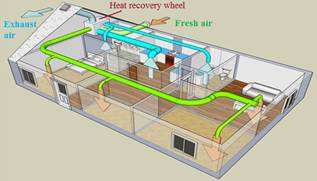Fig. 1. Heat recovery system .

Figure1 shows a typical heat recovery system installed in a ventilation system. In the core, the fresh air stream is automatically preheated or pre-cooled (depending on the season) by the exhausted air and redirected to the cooling, heating or any demanding alternatives. The outgoing and incoming air streams pass next to each other but do not mix in the heat wheel; this providing guaranteed contaminant-free pre-heated or pre-cooled fresh air.

1.2. Heat Recovery Wheel

A thermal wheel consists of a matrix of heat-absorbing material, Fig. 2, which is slowly rotated within the supply and exhaust air streams of an air handling system. When cooling the interior of a building, as the thermal wheel rotates, heat is picked up from the fresh air stream in one-half of the rotation, and given up to the exhaust air stream in the other half of the rotation. Thus, heat energy from the hot fresh air stream is transferred to the matrix material and then from the matrix material to the cool exhaust air stream lowering the temperature of the supply air stream by an amount proportional to the temperature differential between air streams, or 'thermal gradient', and depending upon the efficiency of the device. Heat exchange is most efficient when the streams flow in opposite directions (counter flow), since this causes a favorable temperature gradient across the thickness of the wheel.

Because of the nature of thermal wheels in the way that heat is transferred from one stream to another without having to pass directly through or via an exchange medium, the gross efficiencies are usually much higher than that of any other air-side heat recovery system [3,4].Fig. 2. Heat recovery wheel .

The heat recovery wheel consists of air channels and exchanger walls, which contain not only the adsorbent but also the base materials or the binders. The base material of the exchanger wall may be metal or fiberglass. As an example, the matrix pictures of exchanger wheels and the close-up of the front surface are shown in Fig. 3. As can be seen, they may have different values of width (20, 60,100, 200, 400 mm) for the diameter of 350 mm .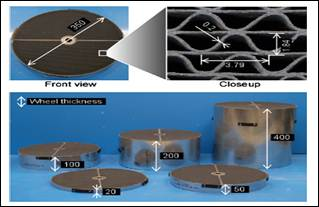Fig. 3. Pictures of heat recovery wheels (mm) .

The major advantages of heat recovery wheel are :

1.   The system significant electrical power savings.

2.   Chlorofluorocarbons (CFCs) free; thus, the system is environmentally friendly.

3.   Construction and maintenance are simple.

2. Background

2.1. Previous Investigations

The design of HVAC (Heating, ventilation and air conditioning) systems for thermal comfort requires increased attention, especially matters arising from recent regulations and standards on ventilation . The optimum temperature and humidity range for human comfort is presented by ASHRAE . Building energy consumption and savings were highlighted by some investigators [9-11]. Much effort was devoted to the research and application of heat conservation wheel components, especially desiccant wheels [2,12,24]. Also, many studies concentrated on the potential use of heat conservation wheel systems in various locations in the USA and Europe [5,6,9,25,28]. Other researchers considered the mathematical models of rotary wheels [20,28,30]. Moreover, artificial neural network (ANN) and adaptive neuro-fuzzy inference system (ANFIS) techniques were used by researchers in air conditioned systems [31-35] and other mechanical applications [36-42].

2.2. Present Work

In the present investigation, a computational study of the channels of heat recovery wheels was carried out for different materials and shapes. The commercial software-package (ANSYS-Fluent 13) was exploited for parametric study of the influence of different parameters on heat transfer performance.

The investigated channels of the heat conservation wheel had five cross-sectional shapes, namely: circular, hexagonal, quadrangular, lozenge and sinusoidal. In addition, four different materials; steel, aluminium, nickel and copper were considered at different values of Reynolds number.

On the other hand, both the artificial neural network (ANN) and adaptive neuro-fuzzy inference system (ANFIS) methods were adapted to predict the important thermal characteristics. The present computational results were used as training and verification data for the artificial intelligence models.

3. Mathematical Model and Computational Features

3.1. Mathematical Model of Flow and Heat

It is assumed that the flow is incompressible, one-dimensional and turbulent through a straight channel, Fig.4. Thermal properties of the fluid (such as specific heat, thermal conductivity, density) are assumed to have bulk average values and be uniform at any cross-section. Mass flow rate is constant. Heat transfer is in the flow direction.

Based on these assumptions, differential equations relating the flow and thermal fields can be derived from the energy balance applied to a unit element through the channel of the heat wheel as a control volume. The details of the mathematical model can be found in [43-45].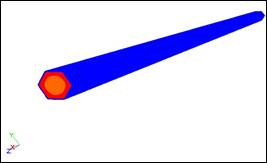Fig. 4. Channel model.

3.2. Turbulence Modeling

The realizable k-e turbulence model was used in the present study. The realizable k-e model differs from the standard k-e model in two important aspects: (i) the realizable k-e model contains an alternative formulation for the turbulent viscosity. (ii) A modified transport equation for the dissipation rate,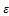, has been derived from an exact equation for the transport of the mean-square vorticity fluctuation. Further details about the realizable k-turbulence model are found in .

3.3. Computational Domain and Boundary Conditions

The conservation wheel contains flow passages that form a matrix of similar channels. The present study concerns the flow and thermal fields through these channels. As these channels are similar, only one complete channel is considered. The flow was treated as steady without the effect of wheel rotation. The flow in the channel was kept hotter than the surroundings.

The computational domain, for all test cases, is a three dimensional (3-D) domain, Fig. 5. The ratio between the length of the channel and its hydraulic diameter (L/d) is (100/3).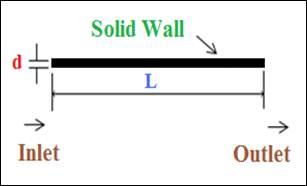Fig. 5. Computational domain and boundary conditions.

Typically, there are three types of boundary conditions that can be listed as:

Inlet boundary condition: inlet uniform velocity is specified at the entrance of the channel. According to ASHRAE handbook 2005 , the inlet temperature was taken as 319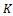. This temperature value represents the annual average ambient temperature in Makkah.

Outlet boundary condition: Pressure outlet flow condition and temperature of 300 K as outlet temperature.

Wall boundary condition: the walls of the channel were treated as smooth. The no-penetration and no-sliding conditions were applied at the channel walls. The temperature at the inner surface of the walls was kept at 309 K for all test cases. This makes a temperature difference of 10 K between the inlet flow and the inner surface. This difference value was recommended in the literature . As will be seen in the following sections, the wall material changes depending on the test case.

3.4. Computational Grid (Mesh)

Due to the complexity of the shapes of the channels, the computational domain was discretized using unstructured grids. This type of grids usually guarantees the flexibility to generate enough computational points in locations of severe gradients. Unstructured grids adopt themselves easily to irregular geometries with minimum programmer's effort.

The computational domain was covered by tetrahedral-shaped elements, (Fig. 6). The grid is very fine next to the solid boundary. The dimensionless distance between the wall and first computational point is y+ ≈ 2. y+ is calculated as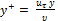. Where, y is the distance to the first point off the wall,is the kinematic viscosity,is the friction velocity (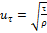),is the wall shear stress, and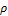is the flow density. The value of y+ = 2 ensures the resolution of the complex turbulent flow [44, 45].

Careful consideration was paid to ensure the grid-independency of the computational solution. Thus, three grid sizes were used to test the grid-independency, namely: 100,000,150,000 and 200,000 elements (cells). The results of both the flow and thermal fields show that the difference between the results of the second and third grid is in the range of 1-2 %. Thus, the second grid size (150,000) was used for all the test cases.Fig. 6. Enlarged grid structure (sample).

3.5. Numerical Treatment

SIMPLE (semi-implicit method for pressure-linked equations) algorithm was employed to solve both the velocity and pressure fields. Each equation of the momentum and energy was solved by "first-order upwind" scheme. The "standard wall function" was used as the near-wall treatment technique in the turbulence model. The solution continues until the numerical residual (error) of all quantities gets below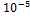.

3.6. Test Cases

A parametric study was carried out to obtain the best case for optimum operation. Different cases were computationally tested and evaluated. The parametric study covered the shape of the channel cross-section, the wall material, and the flow Reynolds number.

Five cross-sectional shapes were considered; circular, hexagonal, quadrangular, lozenge, and sinusoidal. The sinusoidal cross-section is widely used in conservation wheels. It is used here mainly for comparison. The other four shapes are proposed by the authors. The circular shape has good characteristics when considering flow and thermal fields. On the other hand, the hexagonal, quadrangular, and lozenge shapes have good structural properties.

Four materials were tested as wall of the channels, namely: copper, aluminium, nickel and steel. The calculations were performed at six values of Reynolds numbers; 1200, 1540, 1830, 1950, 2200, and 2300. Reynolds number is calculated based on inlet velocity and the hydraulic diameter of the channel, i.e.,. Where,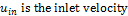,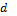is the hydraulic diameter (3 mm),is the kinematic viscosity. This combination of parameters leads to twenty four (24) test cases. Table.1 shows a summary of all the studied test cases.

Table 1. Summary of the test cases.

 Shape Material Reynolds Number CircularCopper 1540 Aluminium 1950 Nickel Steel 2300 Hexagonal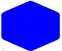Copper 1540 Aluminium 1950 Nickel Steel 2300 Quadrangular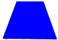Copper 1200 Aluminium 1830 Nickel Steel 2200 Lozenge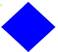Copper 1200 Aluminium 1830 Nickel Steel 2200 Sinusoidal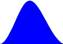Copper 1200 1540 Aluminium 1830 Nickel 1950 Steel 2200 2300

3.7. Validation of the Numerical Results

The present numerical results compare very well to the data obtained by others  as shown in Fig. 7 for the temperature contours. Generally, the same trend of temperature distribution and gradient is obtained in both Figs. 7a and 7b. The red color means the highest temperature and the blue color means the lowest temperature. More gradient contours appear in Fig. 7b. This may be attributed to the secondary flow that is not considered in the present computational investigation.Fig. 7a. Present computational predictions.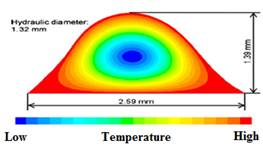Fig. 7b. Results of others .

Fig. 7. Cross-sectional view of the temperature field.

4. Computational Results and Discussions

This section demonstrates the results of the computations of the test cases that were mentioned in Sec. 3.6 and Table 1. The carried-out parametric study aims to find the combination of parameters that gives the optimum performance of the wheel. Optimum performance, here, means best heat transfer exchange rate. However, the results must cover both the flow and thermal fields. The flow filed concerns the working fluid (air) that transfers heat. Thus, the flow field of the different cases is presented firstly. Then, the thermal filed results are presented. Naturally, concentration is paid to the thermal filed as heat transfer optimization is the principal goal of the study.

4.1. Flow Field

Figure 8 shows the velocity contours along the channels as well as at the entrance and exit of the channel for different cross-sections (shapes) and Reynolds number (Re) with aluminium wall. It is clear from the velocity contours at exit that the flow becomes fully-developed before the channel exit. For non-circular cross-sections, the highest velocity is seen in the middle of the channel cross-section. The same pattern is repeated typically for all test cases in Fig. 8. This ensures the fully-developed nature of the flow inside the channel. However, the entrance length may vary from one case to another. This variation of entrance length affect greatly the thermal filed as will be explained in the coming sections.

Figure 9 shows the pressure distribution along the channels as well as at entrance and exit for different cross-sections (shapes) and Reynolds number (Re) with aluminium wall. It is clear from Fig. 9 that the flow in the channel causes a considerable pressure drop from the entrance to the exit. The same pattern is repeated typically for all test cases in Fig. 9.Fig. 8a. Circular, Re = 1950.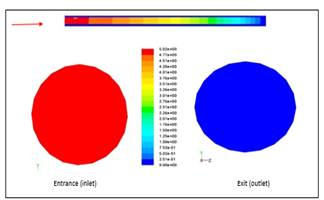Fig. 9a. Circular, Re = 1950.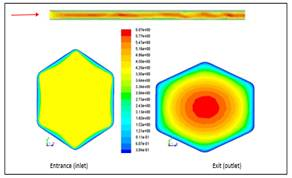Fig. 8b. Hexagonal, Re = 1950.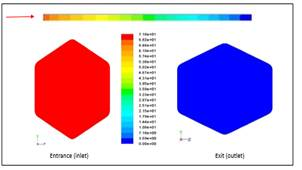Fig. 9b. Hexagonal, Re = 1950.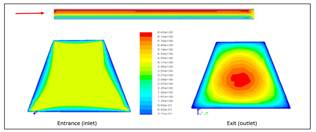Fig. 8c. Quadrangular, Re = 1830.Fig. 9c. Quadrangular, Re = 1830.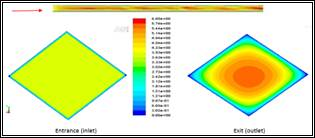Fig. 8d. Lozenge, Re = 1830.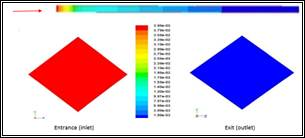Fig. 9d. Lozenge, Re = 1830.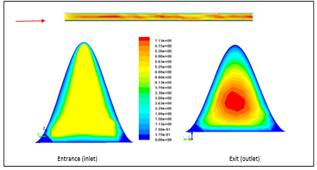Fig. 8e. Sinusoidal, Re = 1830, 1950,.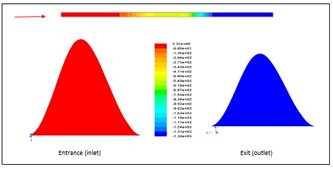Fig. 9e. Sinusoidal, Re = 1830, 1950. Fig. 8. Velocity contours, aluminium wall. Fig. 9. Pressure contours, aluminium wall.

4.2. Thermal Field

To estimate the effect of different parameters on the heat transfer along the channel a certain criterion should be established. This criterion concerns the different thermal zones along the channel in the streamwise direction. Thus, the length of the channel was divided into three consequence zones; hot, middle and cold. For generality the lengths of the three zones are normalized by the total length of the channel. Hence, these lengths can be defined as, Fig. 10:

Hot zone percentage relative length:Middle (Transitional) zone percentage relative length: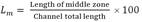Cold zone percentage relative length: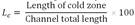Apparently, the greater the value of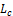, the better the heat transfer process is. This criterion was implemented in the present study to determine the combination of parameters that gives the optimum heat transfer process.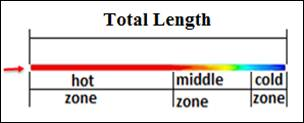Fig. 10. Thermal zones along the channel length.

Figure 11 shows the temperature results for the different cross-sections (shapes) at corresponding values of Reynolds number with various wall materials. The results cover temperature contours as well as the relative lengths of the three thermal zones along the channels.

The first column of Fig. 11 shows the temperature contours along the channel as well as at entrance and exit sections at the corresponding Reynolds number and aluminium wall. Generally, the temperature inside the channel drops from the inlet temperature to the wall temperature well-before exiting the channel. The pattern of temperature contours at entrance and exit of the channel is repeated for all cases. However, the temperature contours along the channel length changes from one case to another depending on the case parameters. The second column of Fig. 11 shows the temperature contours along the channel length for all cases. Based on the results of the second column of Fig. 11, the percentage relative lengths of the thermal zones along the channel for different cases are shown in the third column of Fig. 11.

Figure 11a shows the results of the temperature contours along the channel as well as at entrance and exit sections for the circular cross-section. It is clear that Lc represents most of the length of the channel for all cases. The maximum value of Lc is 0.91 at Re = 1540 with aluminium wall. Hence, the circular shape gives a noticeable good thermal performance.

Figure 11b shows the results for the hexagonal cross-section. Figure 11b(iii) shows that, at the same Reynolds number of 1950, the longest cold zone has a percentage relative lengths of Lc = 25% and Lh = 59% for the copper material. When considering aluminium as the wall material at different values of Reynolds number, the longest cold zone has a percentage relative lengths of Lc = 31% and Lh = 58% at Re = 1540.

Figure 11c shows the results for the quadrangular cross-section. It is clear from Fig. 11c(iii) that, at the same Reynolds number of 1830, the longest cold zone has a percentage relative length of Lc = 94% with corresponding length of hot zone Lh = 3% for the copper material. When considering aluminium as the wall material at different values of Reynolds number, the longest cold zone has a percentage relative length of Lc = 95% with corresponding length of hot zone Lh = 3% at Re = 1200.

Figure 11d shows the results for the Lozenge cross-section. It is obvious from Fig. 11d(iii) that, at the same Reynolds number of 1830, the longest cold zone has a percentage relative length of Lc = 21% with corresponding length of hot zone Lh=71% for copper. When considering aluminium as the wall material at different values of Reynolds number, the longest cold zone has a percentage relative length Lc = 75% with corresponding length of hot zone Lh = 15% at Re = 1200.

Figure 11e shows the results for the sinusoidal cross-section at Re = 1830 and 1950. Figure 11e(iii) illustrates that, at the same Reynolds numbers of 1830 and 1950, the longest cold zone has a percentage relative length of Lc = 65% with corresponding length of hot zone Lh = 18% for copper. When considering aluminium as the wall material at different values of Reynolds number, the longest cold zone has a percentage relative length of Lc = 88% with corresponding length of hot zone Lh = 7% at Re = 1200 and 1540, while for Re = 2200, Lc = 35% and Lh = 45%.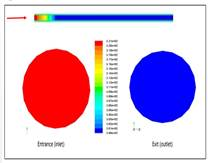Fig. 11a(i). Temperature contours, Re=1950, aluminium wall.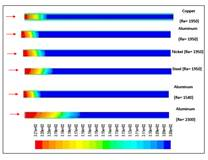Fig. 11a(ii). Temperature contours for all materials.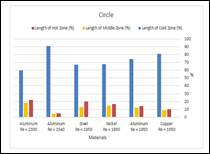Fig. 11a(iii). Bar chart for identifying the three zones. Fig. 11a. Circular cross-section.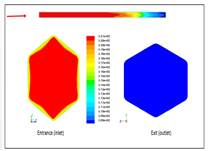Fig. 11b(i). Temperature contours, Re=1950, aluminium wall.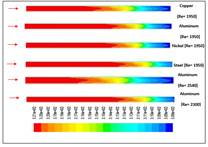Fig. 11b(ii). Temperature contours for all materials.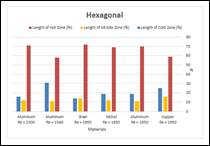Fig. 11b(iii). Bar chart for identifying the three zones. Fig. 11b. Hexagonal cross-section.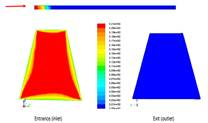Fig. 11c(i).Temperature contours, Re=1830, aluminium wall.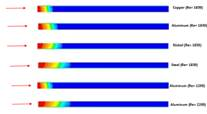Fig. 11c(ii). Temperature contours for all materials.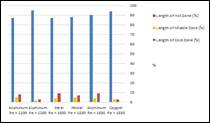Fig. 11c(iii). Bar chart for identifying the three zones. Fig. 11c. Quadrangular cross-section.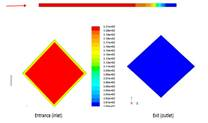Fig. 11d(i). Temperature contours, Re=1830, aluminium wall.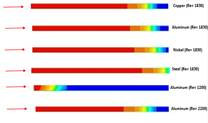Fig. 11d(ii). Temperature contours for all materials.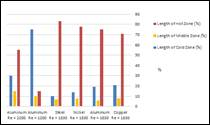Fig. 11d(iii). Bar chart for identifying the three zones. Fig. 11d. Lozenge cross-section.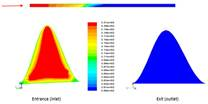Fig. 11e(i). Temperature contours, Re=1830, 1950, aluminium wall.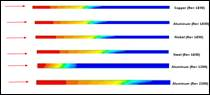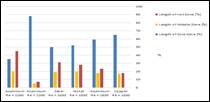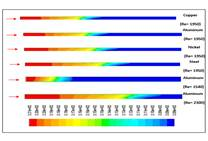Fig. 11e(ii). Temperature contours for all materials.Fig. 11e(iii). Bar chart for identifying the three zones. Fig. 11e. Sinusoidal cross-section. Fig. 11. Temperature results.

4.3. Overall View of All Cases

Based on the results of the previous section an overall view of all cases can be demonstrated. Table 2 illustrates overall results of the percentage relative lengths of all cases.

Table 2. Overall study results.

 Shape Material Length of Cold Zone (%) Length of Middle Zone (%) Length of Hot Zone (%) Circular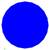Copper Re = 1950 81 9 10 Aluminium Re = 1950 74 12 14 Nickel Re = 1950 68 15 17 Steel Re = 1950 67 13 20 Aluminium Re = 1540 91 4 5 Aluminium Re = 2300 60 18 22 Hexagonal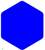Copper Re = 1950 25 16 59 Aluminium Re = 1950 19 11 70 Nickel Re = 1950 19 12 69 Steel Re = 1950 14 14 72 Aluminium Re = 1540 31 11 58 Aluminium Re = 2300 16 12 71 Quadrangular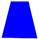Copper Re = 1830 94 3 3 Aluminium Re = 1830 90 4 9 Nickel Re = 1830 88 5 7 Steel Re = 1830 87 4 9 Aluminium Re = 1200 95 2 3 Aluminium Re = 2200 87 5 8 Lozenge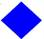Copper Re = 1830 21 8 71 Aluminium Re = 1830 19 6 75 Nickel Re = 1830 14 8 78 Steel Re = 1830 10 7 83 Aluminium Re = 1200 75 10 15 Aluminium Re = 2200 30 15 55 Sinusoidal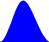Copper Re = 1830, 1950 65 17 18 Aluminium Re = 1830, 1950 59 18 23 Nickel Re = 1830 52 20 28 Steel Re = 1830, 1950 50 19 31 Aluminium Re = 1200, 1540 88 5 7 Aluminium Re = 2200, 2300 35 20 45

From Table 2, the value of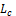decreases with Reynolds number. Thus, decreasing the value of Reynolds number improves the heat transfer process. However, the value of Reynolds number is controlled by the actual operating conditions of the heat recovery wheel. In addition, at the same Reynolds number, copper shows the best heat transfer performance. Nevertheless, copper is more expensive than aluminium.

Generally, the "Circular" and "Quadrangular" are the two shapes that give the best heat transfer response in comparison to the "Sinusoidal" shape, which is commonly used. However, they may be not considered as the optimum choice from construction point of view.

It is concluded that the optimum thermal cooling cases can be stated as:

1.   When considering the same range of Reynolds number (1830-1950) with different materials: Re = 1830, copper wall, quadrangular shape, Lc = 94%.

2.   When considering the same material (aluminium) with different Reynolds numbers: Re = 1200, quadrangular shape, Lc = 95%.

5. Artificial Intelligence (AI)

Both the two artificial intelligence techniques (AI); artificial neural network (ANN) and adaptive neuro-fuzzy inference system (ANFIS) were used to predict the heat transfer performance of the present cases. This strategy was intended to demonstrate and judge the ability of both ANN and ANFIS to predict the heat transfer performance. Moreover, the advantages/disadvantages of the two AI techniques can be clearly noticed. Thus, the predictions of the investigated cases were divided between the two AI techniques. The results of the present computations were used as input data to the two AI techniques in their training phase.

5.1. Artificial Neural Network (ANN)

An ANN was used to predict the percentage relative length Lc for different materials (copper, aluminium, nickel, steel), different Reynolds numbers (1540, 1950, 2300), and different shapes (circular, hexagonal, sinusoidal). A robust learning heuristic for multi-layered feed-forward neural network, called the Generalized Delta Rule (GDR) or "Back Propagation Learning Rule", was implemented in the present work. The back propagation learning rules are used to adjust the weights and biases of ANNs to minimize the sum-squared error of the network. This is done by continually changing the values of the network weights and biases in the direction of steepest descent with respect to error. The used back propagation of a multilayer ANN is shown in Fig. 12.

The neural network toolbox of the Matlab 7.6 package was chosen to train the ANN . The input data comprises three groups (vectors) of data, which are wall materials, Reynolds numbers, and shapes. The output data comprises one group (vector) of data, which is Lc. The computational input and output data were used to train the ANN till it can approximate a function that associates input vectors with specific output. The initial weights as well as the initial biases employed random values between 1 and -1. The nodes in the hidden layer were varied from 2 to 18 for every input pattern and the evaluation of the performance of the network in determining the optimum hidden nodes was carried out. Also, many training cases were operated to get the optimum transfer functions arrangement.

ANN was trained for eighteen different cases. After the ANN was trained, a separate set of unseen test data was supplied as input to the ANN and its performance was evaluated. Table 3 shows the input and the output for each training case. Results for many training cases that were carried out are presented in Table 4. These results are obtained after 1000 epochs. The epoch is a complete iteration of ANN.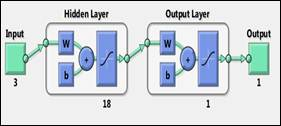Fig. 12. Back propagation of two-layer network with one output layer and one hidden layer .

Table 3. ANN training cases.

 Case 1 2 3 4 5 6 7 8 9 10 11 12 13 14 15 16 17 18 Shape Circular Hexagonal Sinusoidal Input-1 0.1 0.1 0.1 0.1 0.1 0.1 0.2 0.2 0.2 0.2 0.2 0.2 0.3 0.3 0.3 0.3 0.3 0.3 Input-2 1 2 3 4 2 2 1 2 3 4 2 2 1 2 3 4 2 2 Input-3 1950 1950 1950 1950 1540 2300 1950 1950 1950 1950 1540 2300 1950 1950 1950 1950 1540 2300 Output 81 74 68 67 91 60 25 19 19 14 31 16 65 59 52 50 88 35

Key: Input-1 (Shape): (0.1) Circular, (0.2) Hexagonal, (0.3) Sinusoidal. Input-2 (Material): (1) Copper, (2) Aluminium, (3) Nickel, (4) Steel. Input-3 (Reynolds number): 1540, 1950, 2300. Output: Lc.

Table 4. Results of ANN test cases.

 CASE F1 F2 S1 SSE SSET Circular Logsig Purelin 6 0.0573 0.0734 Hexagonal Logsig Purelin 6 0.0607 0.0764 Sinusoidal Logsig Purelin 6 0.0418 0.0697 All Shapes Logsig Purelin 18 0.0217 0.0415

Key: F1: Transfer function of the first layer. F2: Transfer function of the second layer. S1: Number of neurons in the hidden layer. SSE: Sum-squared error for training vector. SSET: Sum-squared error for testing vector.

It is obvious from table 4 that the increase of number of neurons in the hidden layer (S1) enhances the performance of ANN. Thus, when training cases of "All Shapes" are considered, the lowest values of errors (SSE, SSET) are obtained. The predictions of Lc based on new set of input data that has not been used in the training of the neural networks are shown in Table 5.

Figure 13 shows a comparison between computational results and ANN predictions. Based on the results (outputs) of Table 5, Fig. 13, and the previous Tables 3 and 4, it can be said with confidence that the predictions of Table 5 are very good and acceptable. Hence, ANNs are attractive tools for quick and reliable prediction.

Table 5. New input predictions.

 Inputs Output Material Reynolds number Channel shape Lc (%) 2 1600 0.3 84 2 2000 0.3 56 1 2000 0.3 61 2 1600 0.2 27 2 1850 0.2 22 1 1850 0.2 28 2 1440 0.1 93 2 1850 0.1 77 1 2000 0.1 78

Key: Material: (1) Copper, (2) Aluminium, (3) Nickel, (4) Steel. Reynolds number: 1540, 1950, 2300. Channel shape: (0.1) Circle, (0.2) Hexagonal, (0.3) Sinusoidal.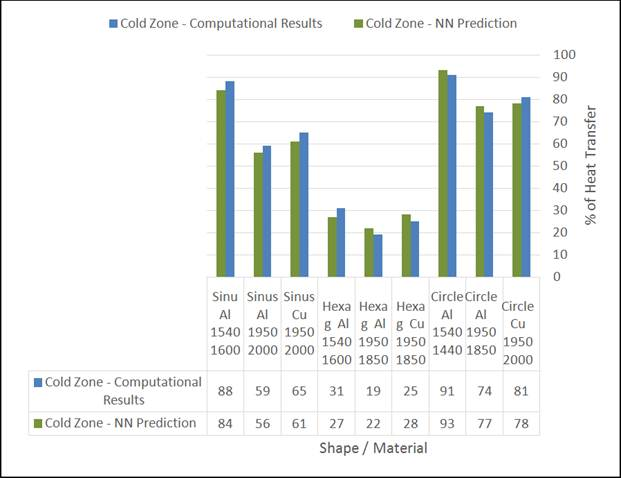Fig. 13. Comparison between computational results and ANN predictions.

5.2. Adaptive Neuro-Fuzzy Inference System (ANFIS)

The basic structure of the fuzzy inference system (FIS), Fig. 14, is a model that maps input characteristics to input membership functions (MF), input membership function to rules, rules to a set of output characteristics, output characteristics to output membership functions (MF), and the output membership functions to a single-valued output or a decision associated with the output .

The parameters (e.g., material, Reynolds number, and channel shape) associated with a given membership function could be chosen so as to tailor the membership functions to a collection of input/output data in order to account for the variations in the data values. This is where the so-called neuro-adaptive learning techniques can help. These techniques provide a method for the fuzzy modeling procedure to learn information about a data set in order to compute the best membership function parameters.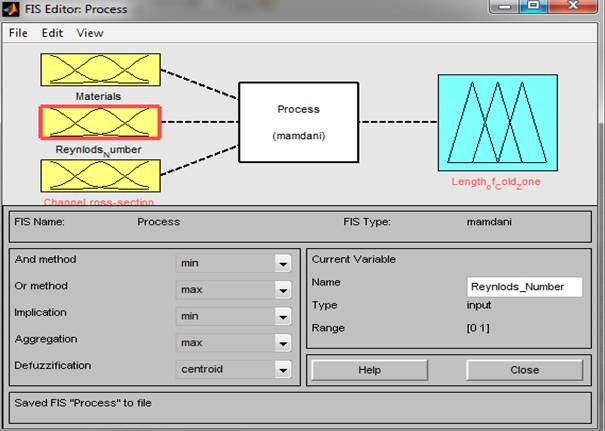Fig. 14. Structure of fuzzy inference system (FIS).

In the present study, four ANFIS models were developed to predict the performance of a heat recovery wheel in terms of its heat transfer efficiency (output) using the toolbox of Matlab 7.6 . The predictions were based on the present computational results. There are three input sets to the ANFIS predictors, namely: material (Input 1), Reynolds number (Input 2), and channel shape (Input 3). The output of the ANFIS is the length of cold zone (Lc). Table 6 shows the input and the output for each training case. The different parameters of the ANFIS models are given in Table 7. All the four ANFIS models were trained until the sum of the squared error reaches 0.006. Thirty epochs were sufficient for the four models.

Table 6. ANFIS training cases.

 Case 1 2 3 4 5 6 7 8 9 10 11 12 13 14 15 16 17 18 Shape Quadrangular Lozenge Sinusoidal Input-1 1 2 3 4 2 2 1 2 3 4 2 2 1 2 3 4 2 2 Input-2 1830 1830 1830 1830 1200 2200 1830 1830 1830 1830 1200 2200 1830 1830 1830 1830 1200 2200 Input-3 0.4 0.4 0.4 0.4 0.4 0.4 0.5 0.5 0.5 0.5 0.5 0.5 0.6 0.6 0.6 0.6 0.6 0.6 Output 94 90 88 87 95 87 21 19 14 10 75 30 65 59 52 50 88 35

Key: Input-1 (Materials): (1) Copper, (2) Aluminium, (3) Nickel, (4) Steel. Input-2 (Reynolds number): 1200, 1830, 2200. Input-3 (Channel shape): (0.4) Quadrangular, (0.5) Lozenge, (0.6) Sinusoidal. Output: Lc

Table 7. Different parameters for the four ANFIS models.

 ANFIS Model Number of MF (Inputs) Input MF type Output MF type Rules of Fuzzy Optimization Method No. of Epochs Quadrangular 2 ´ 6 Gaussmf Linear Grid Partition Hybrid 30 Lozenge 2 ´ 6 Gaussmf Linear Grid Partition Hybrid 30 Sinusoidal 2 ´ 6 Gaussmf Linear Grid Partition Hybrid 30 All Shapes 3 ´ 18 Gaussmf Linear Grid Partition Hybrid 30

The structure of the ANFIS model for quadrangular shape (as an example of the four ANFIS models) is shown in Fig. 15. The output surface of performance, Fig. 16, explains the variation of quadrangular predictions with the three inputs (material, channel shape, Reynolds number).

After ANFIS had been trained, it was tested by checking its predictions by a set of data that had not been seen by ANFIS before, Table 8. The comparison between present computational results and ANFIS predictions for values of Lc is shown in Fig. 17.

When comparing the results (output) of Table 8 and Fig. 17, with the training Table 6, it is clear that the results (output) of Table 8 was very reasonable and well-accepted. Thus, ANFIS proves to be an effective and easy-to-use prediction technique.Fig. 15. Structure of the ANFIS model (quadrangular prediction).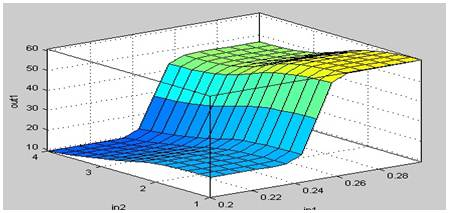Fig. 16. Output surface of performance of the ANFIS model (quadrangular prediction).

Table 8. New input predictions.

 Inputs Output Material Reynolds number Channel shape Lc (%) 2 1300 0.6 82 2 1750 0.6 61 1 1900 0.6 62 2 1100 0.4 96 2 1750 0.4 92 1 1750 0.4 96 2 1300 0.5 70 2 1900 0.5 15 1 1900 0.5 19

Key: Materials: (1) Copper, (2) Aluminium, (3) Nickel, (4) Steel. Reynolds number: 1100, 1300, 1750, 1900. Channel shape: (0.4) Quadrangular, (0.5) Lozenge, (0.6) Sinusoidal.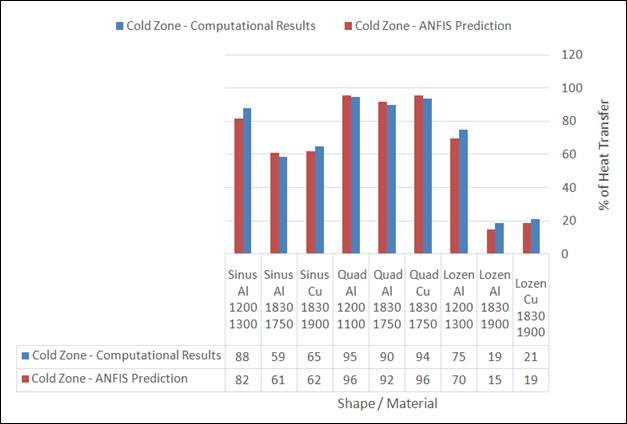Fig. 17. Comparison of heat transfer between present computational results and ANFIS predictions.

6. Conclusions

In the present investigation, a computational study of heat recovery wheel was carried out for different materials and cross-sectional shapes. Also, the Reynolds number of flow was considered as important parameter. The main objective of the study is to find the combination of parameters that give the optimum performance (best heat transfer). The performance was evaluated by modeling the channel flow of the wheels. Also, artificial intelligence techniques (ANN, ANFIS) were proposed to predict the thermal behavior of the investigated cases.

Based on the above discussions, the following concluding points can be stated:

1.   The present computational model and solution scheme were effectively validated. Hence, the outputs of present study are to be considered with confidence.

2.   Generally, the circular and quadrangular shapes are the two shapes that give the finest heat transfer response in comparison to the "Sinusoidal" shape, which is commonly used. However, they may be not considered as the optimum choice from construction point of view.

3.   It can be stated that the optimum thermal cooling cases are as follows:

When considering the same range of Reynolds number (1830-1950) with different materials: Re = 1830, copper material, quadrangular shape, Lc = 94%.

When considering the same material (aluminium) with different Reynolds numbers: Re = 1200, quadrangular shape, Lc = 95%.

4.   Although the hexagonal shape has good structural advantages, it is not the best choice when concerning the thermal performance.

5.   The circular cross-section (shape), which is simple, gives much better thermal performance than the sinusoidal cross-section that is commonly used in heat exchange wheels. However, when using circular channels to construct the recovery wheel, there will be channels between them that are not circular. Thus, the overall performance may deteriorate.

6.   For all cases, the wall materials can be arranged from best to worst as: copper, aluminium, nickel, and steel when considering the thermal performance.

7.   It is very important to state that the above concluding points are based on the stated flow and thermal fields. Other concluding remarks may be obtained for other operating conditions.

8.   The artificial intelligence techniques (ANN, ANFIS) proved to be very effective in predicting the thermal characteristics. They are much easier to program and operate than other computational techniques. However, they need computational and/or experimental data for training.

9.   Energy saving approach adapted in this study guarantees contaminant-free pre-heated or pre-cooled fresh air, which is the major concern in health care facilities and large crowded commercial structures.

Nomenclature

 d : Channel hydraulic diameter F1 : Transfer function of the first layer F2 : Transfer function of the second layer L : Channel length: Cold zone percentage relative length: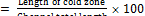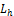: Hot zone percentage relative length: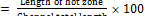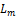: Middle zone percentage relative length: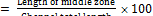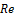: Reynolds number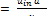S1 : Number of neurons in the hidden layer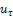: friction velocity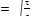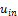: Inlet velocity y : Distance to the first computational point off the wall y+ : Dimensionless distance between the wall and first computational point Greek: Kinematic viscosity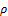: Flow density: Wall shear stress Abbreviations AHU : Air Handling Unit AI : Artificial intelligence ANFIS : Adaptive neuro-fuzzy inference system ANN : Artificial neural network ASHRAE : American Society of Heating, Refrigerating and Air Conditioning Engineers CFC : Chlorofluorocarbons FIS : Fuzzy inference system GDR : Generalized delta rule HVAC : Heating, Ventilation and Air Conditioning MF : Membership functions SIMPLE : Semi-implicit method for pressure-linked equations SSE : Sum-squared error for training vector SSET : Sum-squared error for testing vector

References

1. S. B. Riffat, and G., Gan, "Determination of Effectiveness of Heat-pipe Heat Recovery for Naturally-ventilated Buildings", Applied Thermal Engineering, Vol. 18, No. 3-4, pp. 121-130, 1998.
2. L. A. Sphaier, and W. M. Worek, "Analysis of Heat and Mass Transfer in Porous Sorbents Used in Rotary Regenerators", International Journal of Heat and Mass Transfer, Vol. 47, pp. 3415-3430, 2004.
3. G. J. Softah, Numerical Analysis and Neuro-Fuzzy Investigation of the Performance of Heat Recovery Wheels in AHU Systems, M.Sc. Graduation Project, Mechanical Engineering Department, Umm Al-Qura University, Saudi Arabia, Spring 2014.
4. A. M. Wafiah, Computational and Neural Investigation of the Operation of Heat Exchange Wheels in AHU Systems, M.Sc. Graduation Project, Mechanical Engineering Department, Umm Al-Qura University, Saudi Arabia, Spring 2014.
5. S. Yamaguchi, and K. Saito, "Numerical and Experimental Performance Analysis of Rotary Desiccant Wheels", International Journal of Heat and Mass transfer, Vol. 60, pp. 51-60, 2013.
6. W. Shurcliff, "Air-to-air Heat Exchangers for Houses", Annual Review Energy, Vol. 13, pp. 1-22, 1988.
7. P. Mazzei, F. Minichiello, and D. Palma, "Desiccant HVAC Systems for Commercial Buildings", Applied Thermal Engineering, Vol. 22, No. 5, pp. 545-560, 2002.
8. 2005 ASHRAE Handbook Fundamentals SI Edition, ASHRAE, USA, 2005.
9. L. Pérez-Lombard, J. Ortiz, and C. Pout, "A Review on Buildings Energy Consumption Information", Energy and Buildings, Vol. 40, No. 3, pp. 394-398, 2008.
10. K. K. W. Wan, D. H. W. Li, D. Liu, J. C. Lam, "Future Trends of Building Heating and Cooling Loads and Energy Consumption in Different Climates," Building and Environment, Vol. 46, No. 1, pp. 223-234, 2011.
11. L. Shao, S. B. Riffat, and G. Gan, "Heat Recovery with Low Pressure Loss for Natural Ventilation", Energy and Buildings, Vol. 28, No. 2, pp. 179-184, 1998.
12. A. Pesaran, and A. F. Mills, "Moisture Transport in Silica Gel Packed Bed I: Theoretical Study", International Journal of Heat and Mass Transfer, Vol. 30, pp. 1037-1049, 1987.
13. A. Pesaran, and A. F. Mills, "Moisture Transport in Silica Gel Packed Bed II: Experimental Study", International Journal of Heat and Mass Transfer, Vol. 30, pp. 1051-1060, 1987.
14. W. Zheng, and W. M. Worek, "Numerical Simulation of Combined Heat and Mass Transfer Process in a Rotary Dehumidifier", Numerical Heat Transfer Part A, Vol. 23, pp. 211-232, 1993.
15. Y. J. Dai, R. Z. Wang, and H. F. Zhang, "Parameter Analysis to Improve Rotary Desiccant Dehumidification Using a Mathematical Model", International Journal of Thermal Sciences, Vol. 40 pp. 400-408, 2001.
16. J. L. Niu, and L. Z. Zhang, "Performance Comparisons of Desiccant Wheels for Air Dehumidification and Enthalpy Recovery", Applied Thermal Engineering, Vol. 22, No. 12, pp. 1347-1367, 2002.
17. F. E. Nia, D. V. Paassen, and M. H. Saidi, "Modeling and Simulation of Desiccant wheel for Air Conditioning", Energy and Buildings, Vol. 38, pp.1230-1239, 2006.
18. G. Heidarinejad, H. P. Shahri, and S. Delfani, "The Effect of Geometrical Characteristics of Desiccant Wheel on Its Performance", International Journal of Engineering Transactions B: Application, Vol. 22, No. 1, pp. 63-75, 2009.
19. A. Kodama, T. Hirayama, M. Goto, T. Hirose, and R. E. Critoph, "The Use of Psychometric Charts for the Optimization of a Thermal Swing Desiccant Wheel", Applied Thermal Engineering, Vol. 21, No. 16, pp. 1657-1674, 2001.
20. T. S. Ge, Y. Li, R. Z. Wang, and Y. J. Dai, "A Review of the Mathematical Models for Predicting Rotary Desiccant Wheel, Renew. Sustain. Energy Revs., Vol. 12, No. 6, pp. 1485-1528, 2008.
21. E. M. Sterling, A. Arundel, and T. D. Sterling, "Criteria for Human Exposure to Humidity in Occupied Buildings", ASHRAE Trans., Vol. 91, No. 1, pp. 611-622, 1985.
22. M. H. Ahmed, N. M. Kattab, and M. Fouad, "Evaluation and Optimization of Solar Desiccant Wheel Performance", Renewable Energy, Vol. 30, No. 3, pp. 305-325, 2005.
23. X. J. Zhang, Y. J. Dai, and R. Z. Wang, "A Simulation Study of Heat and Mass Transfer in a Honeycombed Rotary Desiccant Dehumidifier", Applied Thermal Engineering, Vol. 23, No. 8, pp. 989-1003, 2003.
24. L. G. Harriman III, "The Basics of Commercial Desiccant Systems", Heating/Piping/Air Conditioning, Vol. 66, No. 7, pp. 77-85. 1994.
25. H. M. Henning, T. Erpenbeck, C. Hindenberg, and I. S. Santamiria, "The Potential of Solar Energy Use in Desiccant Cooling Cycles", International Journal of Refrigeration, Vol. 24, pp. 220-229, 2001.
26. P. Mavroudaki, C. B. Beggs, P. A. Sleigh, and S. P. Haliiday, "The Potential for Solar Powered Single-stage Desiccant Cooling in Southern Europe", Applied Thermal Engineering, Vol. 22, pp. 1129-1140, 2002.
27. S. P. Halliday, C. B. Beggs, and P. A. Sleigh, "The Use of Solar Desiccant Cooling in the UK: a Feasibility Study", Applied Thermal Engineering, Vol. 22, pp. 1327-1338, 2002.
28. J. Y. San, and S. C. Hsiau, "Effect of Axial Solid Heat Conduction and Mass Diffusion in a Rotary Heat and Mass Regenerator", Int. J. Heat Mass Transfer, Vol. 36, No. 8, pp. 2051-2059, 1993.
29. R. B. Holmberg, "Combined Heat and Mass Transfer in Regenerators with Hygroscopic Materials", ASME J. Heat Transfer, Vol. 101, pp. 205-210, 1979.
30. D. Charoensupaya, and W. M. Worek, "Effect of Adsorbent Heat and Mass Transfer Resistances on Performance of an Open Cycle Adiabatic Desiccant Cooling System", Heat Recov. Sys. CHP, Vol. 8, No. 6, pp. 537-548, 1988.
31. N. Nassif, "Modeling and Optimization of HVAC Systems Using Artificial Neural Network and Genetic Algorithm", Building Simulation, Vol. 7, No. 3, pp. 237-245, June 2014.
32. Z. Li, X. Xu, S. Deng, and D. Pan, "A Novel Neural Network Aided Fuzzy Logic Controller for a Variable Speed (VS) Direct Expansion (DX) Air Conditioning (A/C) System", Applied Thermal Engineering, Vol. 78, pp. 9-23, 2015.
33. B. C. Ng, I. Z. M. Darus, H. Jamaluddin, and H. M. Kamar, "Application of Adaptive Neural Predictive Control for an Automotive Air Conditioning System", Applied Thermal Engineering, Vol. 73, No. 1, pp. 1244-1254, 2014.
34. H. M. Factor, and G. Grossman, "A Packed Bed Dehumidifier/Regenerator for Solar Air Conditioning with Liquid Desiccants", Solar Energy, Vol. 24, No. 6, pp. 541-550, 1980.
35. A. Al-Alili, Y. Hwang, and R. Radermacher, "Review of Solar Thermal Air Conditioning Technologies", International Journal of Refrigeration, Vol. 39, pp. 4-22, 2014.
36. A. F. Abdel Gawad, "Numerical and Neural Study of the Turbulent Flow around Sharp-Edged Bodies", 2002 Joint US ASME-European Fluids Engineering Division Summer Meeting, Montreal, Canada, July 14-18, 2002.
37. O. E. Abdellatif, and A. F. Abdel Gawad, "Experimental, Numerical and Neural Investigation of the Aerodynamic Characteristics for Two-Dimensional Wings in Ground Effect", Al-Azhar Engineering 7th International Conference, Cairo, Egypt, 7-10 April, 2003.
38. A. F. Abdel Gawad, "Study of Both Airflow and Thermal Fields in a Room Using K-eModelling and Neural Networks", Al-Azhar Engineering 7th International Conference, Cairo, Egypt, 7-10 April, 2003.
39. A. F. Abdel Gawad, M. M. Nassief, and N. M. Gurguis, "Numerical and Neural Study of Flow and Heat Transfer Across an Array of Integrated Circuit Components", Journal of Engineering and Applied Science (JEAS), Vol. 52, No. 5, pp. 981-1000, October 2005.
40. A. F. Abdel Gawad, "Computational and Neuro-Fuzzy Study of the Effect of Small Objects on the Flow and Thermal Fields of Bluff Bodies", 8th Biennial ASME conference on Engineering Systems Design and Analysis (ESDA2006), Torino, Italy July 4-7, 2006.
41. A. F. Abdel Gawad, "Investigation of The Dilution of Outfall Discharges Using Computational and Neuro-Fuzzy Techniques", 2007 ASME International Mechanical Engineering Congress & Exposition (IMECE2007), Seattle, Washington, USA, 5-11 November 2007.
42. A. Hosseinzadeh, and M. Karimi, "Prediction of J-Integral Dependence to Residual Stress and Crack Depth on NACA 0012-34 Using FE and ANN", Engineering Solid Mechanics, Vol. 3, No. 2, pp. 103-110, 2015.
43. Z. Wu, R. V. N. Melnik, and F. Borup, "Model-based Analysis and Simulation of Regenerative Heat Wheel", Energy and Buildings, Vol. 38, pp. 502-514, 2006.
44. A. M. Wafiah, A. F. Abdel Gawad, and M. N. Radhwi, "Computational Investigation of the Operation of Heat Conservation Wheels in AHU-Systems", Umm Al-Qura University Journal of Engineering and Islamic Architecture (UQU-UJEA), Vol. 5, No. 2, Ragab 1435 H - May 2014.
45. G. J. Softah, M. N. Radhwi, and A. F. Abdel Gawad, "A Parametric Study of the Performance of Heat Recovery Wheels in HVAC System", Umm Al-Qura University Journal of Engineering and Islamic Architecture (UQU-UJEA), Vol. 5, No. 2, Ragab 1435 H - May 2014.
46. Fluent guide manual, 2011.
47. R. Parsons, ASHRAE Handbook 2005: Fundamentals, American Society of Heating, Refrigeration and Air-Conditioning Engineers.
48. Matlab guide manual, 2011.

 Contents 1. 1.1. 1.2. 2. 2.1. 2.2. 3. 3.1. 3.2. 3.3. 3.4. 3.5. 3.6. 3.7. 4. 4.1. 4.2. 4.3. 5. 5.1. 5.2. 6.
Article ToolsAbstractPDF(1115K)